# 10.8 Vectors  (Page 11/22)

 Page 11 / 22

For the following exercises, evaluate each root.

Evaluate the cube root of $\text{\hspace{0.17em}}z\text{\hspace{0.17em}}$ when $\text{\hspace{0.17em}}z=64\mathrm{cis}\left(210°\right).$

Evaluate the square root of $\text{\hspace{0.17em}}z\text{\hspace{0.17em}}$ when $\text{\hspace{0.17em}}z=25\mathrm{cis}\left(\frac{3\pi }{2}\right).$

$5\mathrm{cis}\left(\frac{3\pi }{4}\right),5\mathrm{cis}\left(\frac{7\pi }{4}\right)$

For the following exercises, plot the complex number in the complex plane.

$6-2i$

$-1+3i$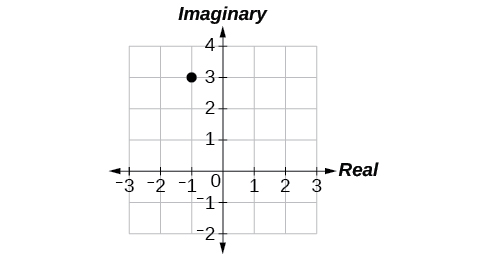## Parametric Equations

For the following exercises, eliminate the parameter $\text{\hspace{0.17em}}t\text{\hspace{0.17em}}$ to rewrite the parametric equation as a Cartesian equation.

$\left\{\begin{array}{l}x\left(t\right)=3t-1\hfill \\ y\left(t\right)=\sqrt{t}\hfill \end{array}$

${x}^{2}+\frac{1}{2}y=1$

Parameterize (write a parametric equation for) each Cartesian equation by using $\text{\hspace{0.17em}}x\left(t\right)=a\mathrm{cos}\text{\hspace{0.17em}}t\text{\hspace{0.17em}}$ and $\text{\hspace{0.17em}}y\left(t\right)=b\mathrm{sin}\text{\hspace{0.17em}}t\text{\hspace{0.17em}}$ for $\text{\hspace{0.17em}}\frac{{x}^{2}}{25}+\frac{{y}^{2}}{16}=1.$

Parameterize the line from $\text{\hspace{0.17em}}\left(-2,3\right)\text{\hspace{0.17em}}$ to $\text{\hspace{0.17em}}\left(4,7\right)\text{\hspace{0.17em}}$ so that the line is at $\text{\hspace{0.17em}}\left(-2,3\right)\text{\hspace{0.17em}}$ at $\text{\hspace{0.17em}}t=0\text{\hspace{0.17em}}$ and $\text{\hspace{0.17em}}\left(4,7\right)\text{\hspace{0.17em}}$ at $\text{\hspace{0.17em}}t=1.$

$\left\{\begin{array}{l}x\left(t\right)=-2+6t\hfill \\ y\left(t\right)=3+4t\hfill \end{array}$

## Parametric Equations: Graphs

For the following exercises, make a table of values for each set of parametric equations, graph the equations, and include an orientation; then write the Cartesian equation.

$\left\{\begin{array}{l}x\left(t\right)=3{t}^{2}\hfill \\ y\left(t\right)=2t-1\hfill \end{array}$

$\left\{\begin{array}{l}x\left(t\right)={e}^{t}\hfill \\ y\left(t\right)=-2{e}^{5\text{\hspace{0.17em}}t}\hfill \end{array}$

$y=-2{x}^{5}$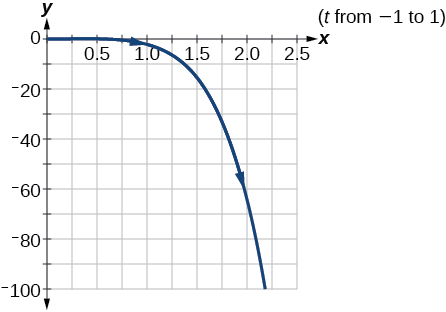$\left\{\begin{array}{l}x\left(t\right)=3\mathrm{cos}\text{\hspace{0.17em}}t\hfill \\ y\left(t\right)=2\mathrm{sin}\text{\hspace{0.17em}}t\hfill \end{array}$

A ball is launched with an initial velocity of 80 feet per second at an angle of 40° to the horizontal. The ball is released at a height of 4 feet above the ground.

1. Find the parametric equations to model the path of the ball.
2. Where is the ball after 3 seconds?
3. How long is the ball in the air?
1. $\left\{\begin{array}{l}x\left(t\right)=\left(80\mathrm{cos}\left(40°\right)\right)t\\ y\left(t\right)=-16{t}^{2}+\left(80\mathrm{sin}\left(40°\right)\right)t+4\end{array}$
2. The ball is 14 feet high and 184 feet from where it was launched.
3. 3.3 seconds

## Vectors

For the following exercises, determine whether the two vectors, $\text{\hspace{0.17em}}u\text{\hspace{0.17em}}$ and $\text{\hspace{0.17em}}v,\text{\hspace{0.17em}}$ are equal, where $\text{\hspace{0.17em}}u\text{\hspace{0.17em}}$ has an initial point $\text{\hspace{0.17em}}{P}_{1}\text{\hspace{0.17em}}$ and a terminal point $\text{\hspace{0.17em}}{P}_{2},\text{\hspace{0.17em}}$ and $\text{\hspace{0.17em}}v\text{\hspace{0.17em}}$ has an initial point $\text{\hspace{0.17em}}{P}_{3}\text{\hspace{0.17em}}$ and a terminal point $\text{\hspace{0.17em}}{P}_{4}.$

${P}_{1}=\left(-1,4\right),{P}_{2}=\left(3,1\right),{P}_{3}=\left(5,5\right)$ and $\text{\hspace{0.17em}}{P}_{4}=\left(9,2\right)$

${P}_{1}=\left(6,11\right),{P}_{2}=\left(-2,8\right),{P}_{3}=\left(0,-1\right)\text{\hspace{0.17em}}$ and $\text{\hspace{0.17em}}{P}_{4}=\left(-8,2\right)$

not equal

For the following exercises, use the vectors $\text{\hspace{0.17em}}u=2i-j\text{,}v=4i-3j\text{,}\text{\hspace{0.17em}}$ and $\text{\hspace{0.17em}}w=-2i+5j\text{\hspace{0.17em}}$ to evaluate the expression.

u v

2 v u + w

4 i

For the following exercises, find a unit vector in the same direction as the given vector.

a = 8 i − 6 j

b = −3 i j

$-\frac{3\sqrt{10}}{10}$ i $-\frac{\sqrt{10}}{10}$ j

For the following exercises, find the magnitude and direction of the vector.

$⟨6,-2⟩$

$⟨-3,-3⟩$

Magnitude: $\text{\hspace{0.17em}}3\sqrt{2},\text{\hspace{0.17em}}$ Direction: $\text{225°}$

For the following exercises, calculate $\text{\hspace{0.17em}}u\cdot v\text{.}$

u = −2 i + j and v = 3 i + 7 j

u = i + 4 j and v = 4 i + 3 j

$\text{16}$

Given v $=〈-3,4〉$ draw v , 2 v , and $\text{\hspace{0.17em}}\frac{1}{2}$ v .

Given the vectors shown in [link] , sketch u + v , u v and 3 v .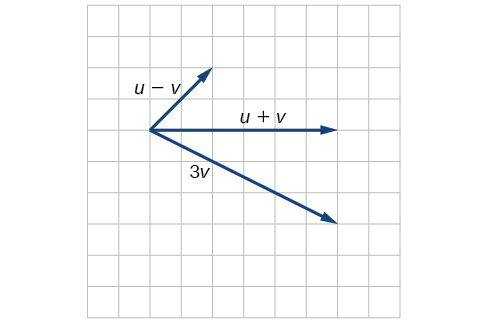Given initial point $\text{\hspace{0.17em}}{P}_{1}=\left(3,2\right)\text{\hspace{0.17em}}$ and terminal point $\text{\hspace{0.17em}}{P}_{2}=\left(-5,-1\right),\text{\hspace{0.17em}}$ write the vector $\text{\hspace{0.17em}}v\text{\hspace{0.17em}}$ in terms of $\text{\hspace{0.17em}}\text{\hspace{0.17em}}i\text{\hspace{0.17em}}\text{\hspace{0.17em}}$ and $\text{\hspace{0.17em}}j.\text{\hspace{0.17em}}$ Draw the points and the vector on the graph.

## Practice test

Assume $\text{\hspace{0.17em}}\alpha \text{\hspace{0.17em}}$ is opposite side $\text{\hspace{0.17em}}a,\beta \text{\hspace{0.17em}}$ is opposite side $\text{\hspace{0.17em}}b,\text{\hspace{0.17em}}$ and $\text{\hspace{0.17em}}\gamma \text{\hspace{0.17em}}$ is opposite side $\text{\hspace{0.17em}}c.\text{\hspace{0.17em}}$ Solve the triangle, if possible, and round each answer to the nearest tenth, given $\text{\hspace{0.17em}}\beta =68°,b=21,c=16.$

$\alpha =67.1°,\gamma =44.9°,a=20.9$

Find the area of the triangle in [link] . Round each answer to the nearest tenth.

A pilot flies in a straight path for 2 hours. He then makes a course correction, heading 15° to the right of his original course, and flies 1 hour in the new direction. If he maintains a constant speed of 575 miles per hour, how far is he from his starting position?

Convert $\text{\hspace{0.17em}}\left(2,2\right)\text{\hspace{0.17em}}$ to polar coordinates, and then plot the point.

Convert $\text{\hspace{0.17em}}\left(2,\frac{\pi }{3}\right)\text{\hspace{0.17em}}$ to rectangular coordinates.

$\left(1,\sqrt{3}\right)$

Convert the polar equation to a Cartesian equation: $\text{\hspace{0.17em}}{x}^{2}+{y}^{2}=5\mathrm{y.}$

Convert to rectangular form and graph: $r=-3\mathrm{csc}\text{\hspace{0.17em}}\theta .$

$y=-3$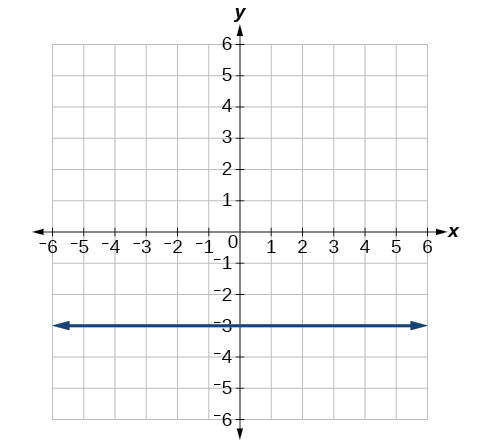Test the equation for symmetry: $\text{\hspace{0.17em}}r=-4\mathrm{sin}\left(2\theta \right).$

Graph $\text{\hspace{0.17em}}r=3+3\mathrm{cos}\text{\hspace{0.17em}}\theta .$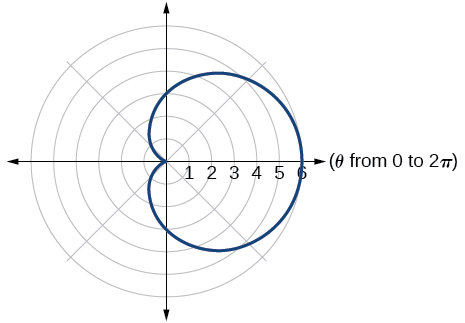Graph $\text{\hspace{0.17em}}r=3-5\text{sin}\text{\hspace{0.17em}}\theta .$

Find the absolute value of the complex number $5-9i.$

$\sqrt{106}$

Write the complex number in polar form: $\text{\hspace{0.17em}}4+i\text{.}$

Convert the complex number from polar to rectangular form: $\text{\hspace{0.17em}}z=5\text{cis}\left(\frac{2\pi }{3}\right).$

$\frac{-5}{2}+i\frac{5\sqrt{3}}{2}$

Given $\text{\hspace{0.17em}}{z}_{1}=8\mathrm{cis}\left(36°\right)\text{\hspace{0.17em}}$ and $\text{\hspace{0.17em}}{z}_{2}=2\mathrm{cis}\left(15°\right),$ evaluate each expression.

${z}_{1}{z}_{2}$

$\frac{{z}_{1}}{{z}_{2}}$

$4\mathrm{cis}\left(21°\right)$

${\left({z}_{2}\right)}^{3}$

$\sqrt{{z}_{1}}$

$2\sqrt{2}\mathrm{cis}\left(18°\right),2\sqrt{2}\mathrm{cis}\left(198°\right)$

Plot the complex number $\text{\hspace{0.17em}}-5-i\text{\hspace{0.17em}}$ in the complex plane.

Eliminate the parameter $\text{\hspace{0.17em}}t\text{\hspace{0.17em}}$ to rewrite the following parametric equations as a Cartesian equation: $\text{\hspace{0.17em}}\left\{\begin{array}{l}x\left(t\right)=t+1\hfill \\ y\left(t\right)=2{t}^{2}\hfill \end{array}.$

$y=2{\left(x-1\right)}^{2}$

Parameterize (write a parametric equation for) the following Cartesian equation by using $\text{\hspace{0.17em}}x\left(t\right)=a\mathrm{cos}\text{\hspace{0.17em}}t\text{\hspace{0.17em}}$ and $\text{\hspace{0.17em}}y\left(t\right)=b\mathrm{sin}\text{\hspace{0.17em}}t:$ $\frac{{x}^{2}}{36}+\frac{{y}^{2}}{100}=1.$

Graph the set of parametric equations and find the Cartesian equation: $\text{\hspace{0.17em}}\left\{\begin{array}{l}x\left(t\right)=-2\mathrm{sin}\text{\hspace{0.17em}}t\hfill \\ y\left(t\right)=5\mathrm{cos}\text{\hspace{0.17em}}t\hfill \end{array}.$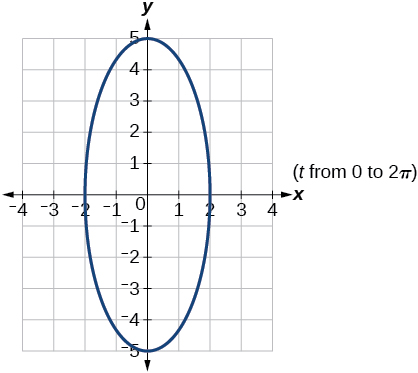A ball is launched with an initial velocity of 95 feet per second at an angle of 52° to the horizontal. The ball is released at a height of 3.5 feet above the ground.

1. Find the parametric equations to model the path of the ball.
2. Where is the ball after 2 seconds?
3. How long is the ball in the air?

For the following exercises, use the vectors u = i − 3 j and v = 2 i + 3 j .

Find 2 u − 3 v .

−4 i − 15 j

Calculate $\text{\hspace{0.17em}}u\cdot v.$

Find a unit vector in the same direction as $\text{\hspace{0.17em}}v.$

$\frac{2\sqrt{13}}{13}i+\frac{3\sqrt{13}}{13}j$

Given vector $\text{\hspace{0.17em}}v\text{\hspace{0.17em}}$ has an initial point $\text{\hspace{0.17em}}{P}_{1}=\left(2,2\right)\text{\hspace{0.17em}}$ and terminal point $\text{\hspace{0.17em}}{P}_{2}=\left(-1,0\right),\text{\hspace{0.17em}}$ write the vector $\text{\hspace{0.17em}}v\text{\hspace{0.17em}}$ in terms of $\text{\hspace{0.17em}}i\text{\hspace{0.17em}}$ and $\text{\hspace{0.17em}}j.\text{\hspace{0.17em}}$ On the graph, draw $\text{\hspace{0.17em}}v,\text{\hspace{0.17em}}$ and $\text{\hspace{0.17em}}-v.\text{\hspace{0.17em}}$

A laser rangefinder is locked on a comet approaching Earth. The distance g(x), in kilometers, of the comet after x days, for x in the interval 0 to 30 days, is given by g(x)=250,000csc(π30x). Graph g(x) on the interval [0, 35]. Evaluate g(5)  and interpret the information. What is the minimum distance between the comet and Earth? When does this occur? To which constant in the equation does this correspond? Find and discuss the meaning of any vertical asymptotes.
The sequence is {1,-1,1-1.....} has
how can we solve this problem
Sin(A+B) = sinBcosA+cosBsinA
Prove it
Eseka
Eseka
hi
Joel
June needs 45 gallons of punch. 2 different coolers. Bigger cooler is 5 times as large as smaller cooler. How many gallons in each cooler?
7.5 and 37.5
Nando
find the sum of 28th term of the AP 3+10+17+---------
I think you should say "28 terms" instead of "28th term"
Vedant
the 28th term is 175
Nando
192
Kenneth
if sequence sn is a such that sn>0 for all n and lim sn=0than prove that lim (s1 s2............ sn) ke hole power n =n
write down the polynomial function with root 1/3,2,-3 with solution
if A and B are subspaces of V prove that (A+B)/B=A/(A-B)
write down the value of each of the following in surd form a)cos(-65°) b)sin(-180°)c)tan(225°)d)tan(135°)
Prove that (sinA/1-cosA - 1-cosA/sinA) (cosA/1-sinA - 1-sinA/cosA) = 4
what is the answer to dividing negative index
In a triangle ABC prove that. (b+c)cosA+(c+a)cosB+(a+b)cisC=a+b+c.
give me the waec 2019 questionsByByByByBy